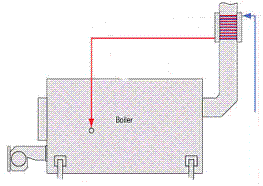# Compare Heat Pump to Condensing Boiler

In our articles Geothermal Heat Pump, and Air Source Heat Pump we looked at how heat pumps can be used to heat water (and air) in a building in an efficient manner.The typical boiler installed in the vast majority of new homes (and now retrofitted to old homes) is the condensing boiler (aka condenser boiler) – a very energy efficient type of gas-powered boiler which runs typically at around 90% efficiency. A condening boiler uses the heat from the flue gases to pre-heat the water before it enters the boiler – thereby reducing gas consumption. Gas boilers installed before 1990 were typically 60% or less efficient, so changing an old boiler to a condensing boiler could knock 30+% off your gas bills.

### Heat Pump or Condensing Boiler?

With the latest gas powered boilers being so efficient, which is better to use – an air source or ground source heap pump, or a condensing boiler?

In the article Air Source Heat Pump we introduced the CoP – the Coefficient of Performance. This is a measure of the number of units of heating obtained for each unit of electricity consumed by the heat pump. We can work out the CoP at which it makes economic and environmental sense to use a heat pump (the break even point), and then install a heat pump with a CoP greater than this value in order to reduce carbon emissions and heating costs.

At break-even point the CO2 emissions per kWh unit of gas (0.193 kg / kWh) divided by the efficiency of the condensing boiler will be equal to the CO2 emissions per kWh unit of electricity (0.432 kg / kWh) divided by the efficiency (CoP) of the heat pump. Therefore, the break-even point for emissions (assuming a 90% efficient condensing boiler) is:

0.90 * (0.432 / 0.193) = 2.24 CoP

Therefore, if an air-source (or ground-source) heat pump has a CoP of greater than 2.24, it will release less carbon emmissions than a condensing boiler.

A similar calculation can be carried out on the cost benefits of a heat pump over a condensing boiler, and (at UK prices where gas is less than half the price of electricity per kWh), the break-even CoP is also around 2.2-2.3, i.e. a heat pump with a CoP of > 2.3 will reduce fuel bills.

### The Effect of Ambient Temperature on CoP

The CoP of an air source heat pump depends on the temperature of the air outside. The colder it is outside, the more electricity is consumed extracting useful heat from it. On a typical mild British spring morning, a typical air-source heat pump will have a CoP of between 3 and 4. At around -15 degrees the CoP will fall below 1 – i.e. it will take more than 1 unit of electricity to generate 1 unit of heat.

The temperature at which a heat pump has a CoP less than the 2.3 break-even point calculated earlier is around 0 degrees Celcius – therefore, in the UK where extended periods with air temperatures below zero are rare, a typical air-source heat pump will generate less carbon emissions and cost less money to run than the best condensing boiler.

### The Final Advantage of a Heat Pump

While the latest gas condensing boilers are ultra-efficient, they depend upon finite fossil fuels. The heat extracted from the air outside (or the ground) by a heat pump is generated by solar power from the Sun’s rays shining on the Earth, and is therefore ‘green’. If electricity generated from renewable sources such as PV solar and wind is used to power a heat pump (e.g. by changing your electricity supplier) then your home and water can be heated entirely renewably (and cost effectively).